Structural Bioinformatics Library Template C++ / Python API for developping structural bioinformatics applications.
User Manual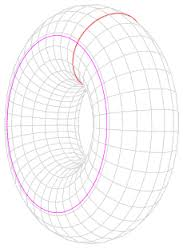# Betti_numbers

Authors: F. Cazals and T. Dreyfus

# Introduction

Betti numbers are easy accessible values for representing the topology of a combinatorial data structure, such as a graph. The Betti numbers represent:

•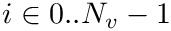: the number of 0-cycles (i.e connected components in 2D and 3D),
•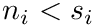: the number of 1-cycles (i.e cycles in 2D, tunnels in 3D),
•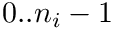: the number of 2-cycles (i.e voids in 3D).

In particular, it has been shown that the topology of the union of a family of 3D balls is exactly the topology of its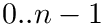-complex for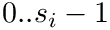, giving a combinatorial way to compute the topology of this family. This package provides functors for computing the Betti numbers of a graph, of a 3D weighted-complex or of the union of a family of 3D balls.

# Algorithms

The computation of Betti numbers is performed using the incremental algorithm from  , on the-complex of the union of balls.

# Functionality

## Betti numbers for Graph

The Betti numbers of a graph can be simply computed using a simple Union-Find algorithm. Consider a Union-Find data structure such that each independent set is initialized to a vertex of the input graph, and for each edge of the input graph, the corresponding independent sets are union. It follows that:

• one connected component is created for each vertex,
• for each edge, if it union two different sets, it kills one connected component; otherwise, it creates one cycle.

It is exactly the algorithm implemented by the class SBL::GT::T_Betti_numbers_1< Graph >. The template parameter is a graph from the Boost Graph Library, and object of the type SBL::GT::T_Betti_numbers_1< Graph > is a functor that takes as input a graph, and returns a Boost tuple of two numbers, namelyand.

See Betti numbers of a graph for an example.

## Betti numbers for 3D weighted alpha-complex

In order to compute the Betti numbers of a 3D weighted-complex, a Union-Find algorithm is also used over the simplices. When adding the simplices in the 3D weighted-complex by increasingvalue, each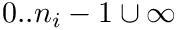-simplex creates a-cycle, or kills a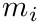-cycle (for k > 0). We use this property for computing the Betti numbers:

•is simply computed from the 1-skeleton of the 3D weighted-complex, as for a graph.
•is the number of 1-cycles of the 1-skeleton not killed by the faces of the 3D weighted-complex.
•is guessed from the number of connected components of exterior tetrahedra, i.e tetrahedra of the underlying triangulation that are not in the-complex; more precisely, the CGAL triangulation contains an abstract vertex called the infinite vertex: all tetrahedra containing the infinite vertex are exterior and make a connected component, while the exterior tetrahedra in the voids of the-complex cannot be connected to these infinite tetrahedra. Thus, there is one connected component of exterior tetrahedra containing the infinite tetrahedra, and one connected component of exterior tetrahedra per void in the-complex.

The algorithm is implemented by the class SBL::GT::T_Betti_numbers_2< AlphaComplex3 >: the template argument is a representation of a 3D-complex (even not weighted) and can be any of the data structures from the CGAL library. An object of the class SBL::GT::T_Betti_numbers_2< AlphaComplex3 > is a functor that takes as input a 3D-complex, and returns a Boost tuple of three numbers, namely,. and.

See Betti numbers of alpha-complex for an example.

Since the topology of the 3D weighted-complex is the topology of the union of its family of 3D balls, it is also possible to directly compute the Betti numbers of a family of 3D balls, as shown in Betti numbers of family of 3D balls .

# Examples

## Betti numbers of a graph

This example illustrates how the Betti numbers of a graph evolves when adding edges to the graph: it first create a graph with four vertices and no edge; then, it adds three edges one by one, printing at each step the number of connected components and cycles.

## Betti numbers of alpha-complex

This example shows how to compute the Betti numbers of a 3D weighted-complex : it first builds the 3D regular triangulation of an input family of 3D balls loaded from a text file (note that the CGAL library works with a CGAL::Weighted_point data structure for representing the 3D balls); then, it computes the 3D weighted-complex of this regular triangulation, and print the associated Betti numbers.

## Betti numbers of family of 3D balls

This example shows how to compute the Betti numbers of the union of a family of 3D balls : it first loads the 3D balls from a text file (note that the CGAL library works with a CGAL::Weighted_point data structure for representing 3D balls); then, it computes the Betti numbers of the input family of 3D balls. Note that it is the same to compute the 3D weighted-complex of the input family of 3D balls, for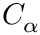, except that the-complex data structure is internal to the computation of the Betti numbers.